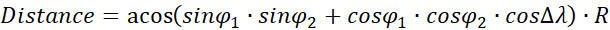SpreadsheetWeb Hub supports Excel's LAMBDA function. Recently released LAMBDA function allows Excel users to build custom, reusable functions without VBA. There are so many use cases of LAMBDA function. In this article, we will build a function to calculate distance between two points represented by their latitudes and longitudes. Then we will build a SpreadsheetWeb application using this function for distance calculation.

Latitude/longitude calculations are always tricky thanks to the ellipsoidal shape of the earth. Although, you accept the earth as perfect sphere like most calculation approaches, the curvy structure works with degrees, minutes, and seconds while distance is measured in decimals. This conversion increases the length of your formula immensely and makes it harder to reuse and manage.

## Formula of calculating distance by longitude and latitude

In our example, we are using the great-circle distance method which uses the shortest path, also known as, as the crow flies, between two points corresponding to an arc linking two points on a sphere. The method uses Spherical law of cosines a theorem relating the sides and angles of spherical triangles.where

• R: Radius of the earth

The coordinates are represented in two forms:

• As a string including degree, minute and second
• As a numerical value of decimal degrees

Converting degrees, minutes and seconds to decimal degrees:

Decimal Degrees = Degrees + (Minutes + Seconds / 60) / 60

Radians = Decimal Degrees * PI / 180

Because deg-min-sec notation will make things more confusing, we will use decimal degrees going forward. This is the Excel formula for decimal degrees:

ACOS(

SIN(Place1_Lat * PI() / 180) *

SIN(Place2_Lat * PI() / 180) +

COS(Place1_Lat * PI() / 180) *

COS(Place2_Lat * PI() / 180) *

COS(Place2_Lon * PI() / 180 - Place1_Lon * PI() / 180)

Obviously, no one wants to deal with this kind of formula more than one time. Instead, you can use the LAMBDA function to define this formula once in a named range and use the named range like a UDF (user-defined function). Let's see what the LAMBDA function is and how you can use it.

LAMBDA Function

The LAMBDA function is game changer feature that allows you create your own functions without any VBA, macro, or JavaScript knowledge. Since it's unique nature, the function has a unique use case.

• A LAMBDA formula should be written in a named range. That named range's name will be your custom function's name.
• You need to type parameter names before the function itself. Such as, a and b are the parameters and the latter operation is the function itself: =LAMBDA(a, b, a-b)
• LAMBDA functions can be called recursively.

LAMBDA([parameter1, parameter2, …,] calculation)

For detailed information, please visit the following page: Excel LAMBDA Function

The LAMBDA function requires a Microsoft 365 subscription.

## Calculating distance by longitude and latitude with LAMBDA Function

Let's create our custom function as described above. The distance formula needs 4 arguments, if we use a static value for the radius of earth, e.g., 6,371 km.

=LAMBDA(

Place1_Lat,

Place1_Lon,

Place2_Lat,

Place2_Lon,

ACOS( SIN(Place1_Lat * PI() / 180) * SIN(Place2_Lat * PI() / 180) + COS(Place1_Lat * PI() / 180) * COS(Place2_Lat * PI() / 180) * COS(Place2_Lon * PI() / 180 - Place1_Lon * PI() / 180) ) * 6371)Once created, you can re-use your custom function anywhere in this workbook. Excel even displays IntelliSense box for this function.Tip: You can use Excel's RADIANS function instead of multiplying by PI() / 180.